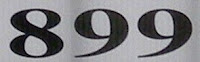## Monday, December 20, 2010

### 899

899 = 29 x 31. It is the product of two successive primes and the product of twin primes.

Any number consisting of an 8 followed by an even number of nines has prime factors 3 x (10n/2) + 1 and 3 x (10n/2) - 1, where n is the number of nines: 899 = 3(101) + 1) x 3(101) - 1 = 31 x 29. Similarly, 8999999 = 2999 x 3001.

899 is the smallest number whose sum of digits is 26.

899 is a number that can't be expressed as the sum of a prime number and a Fibonacci number.The Benneli TNT 899 Sport is an Italian motorcycle.